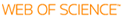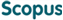### BROWSE

#### Related ResearcherJung, Chang-Yeol
Analysis and computational methods Lab
Research Interests
• Analysis, singular perturbations, uncertainty, numerical methods

Finite Volume Approximation of One-Dimensional Stiff Convection-Diffusion Equations

Cited 0 times inCited 5 times inTitle
Finite Volume Approximation of One-Dimensional Stiff Convection-Diffusion Equations
Author
Keywords
Analytic method; Boundary layer structure; Convection-diffusion equations; Correctors; Discretizations; Finite volume; Finite volume approximation; Finite volume schemes; Novel methods; Second-order scheme; Singularly perturbed problem; Singularly perturbed problems; Stability and convergence; Stiff problem; Up-wind scheme
Issue Date
2009-12
Publisher
SPRINGER/PLENUM PUBLISHERS
Citation
JOURNAL OF SCIENTIFIC COMPUTING, v.41, no.3, pp.384 - 410
Abstract
In this work, we present a novel method to approximate stiff problems using a finite volume (FV) discretization. The stiffness is caused by the existence of a small parameter in the equation which introduces a boundary layer. The proposed semi-analytic method consists in adding in the finite volume space the boundary layer corrector which encompasses the singularities of the problem. We verify the stability and convergence of our finite volume schemes which take into account the boundary layer structures. A major feature of the proposed scheme is that it produces an efficient stable second order scheme to be compared with the usual stable upwind schemes of order one or the usual costly second order schemes demanding fine meshes.
URI
DOI
10.1007/s10915-009-9304-2
ISSN
0885-7474
Appears in Collections:
SNS_Journal Papers
Files in This Item: